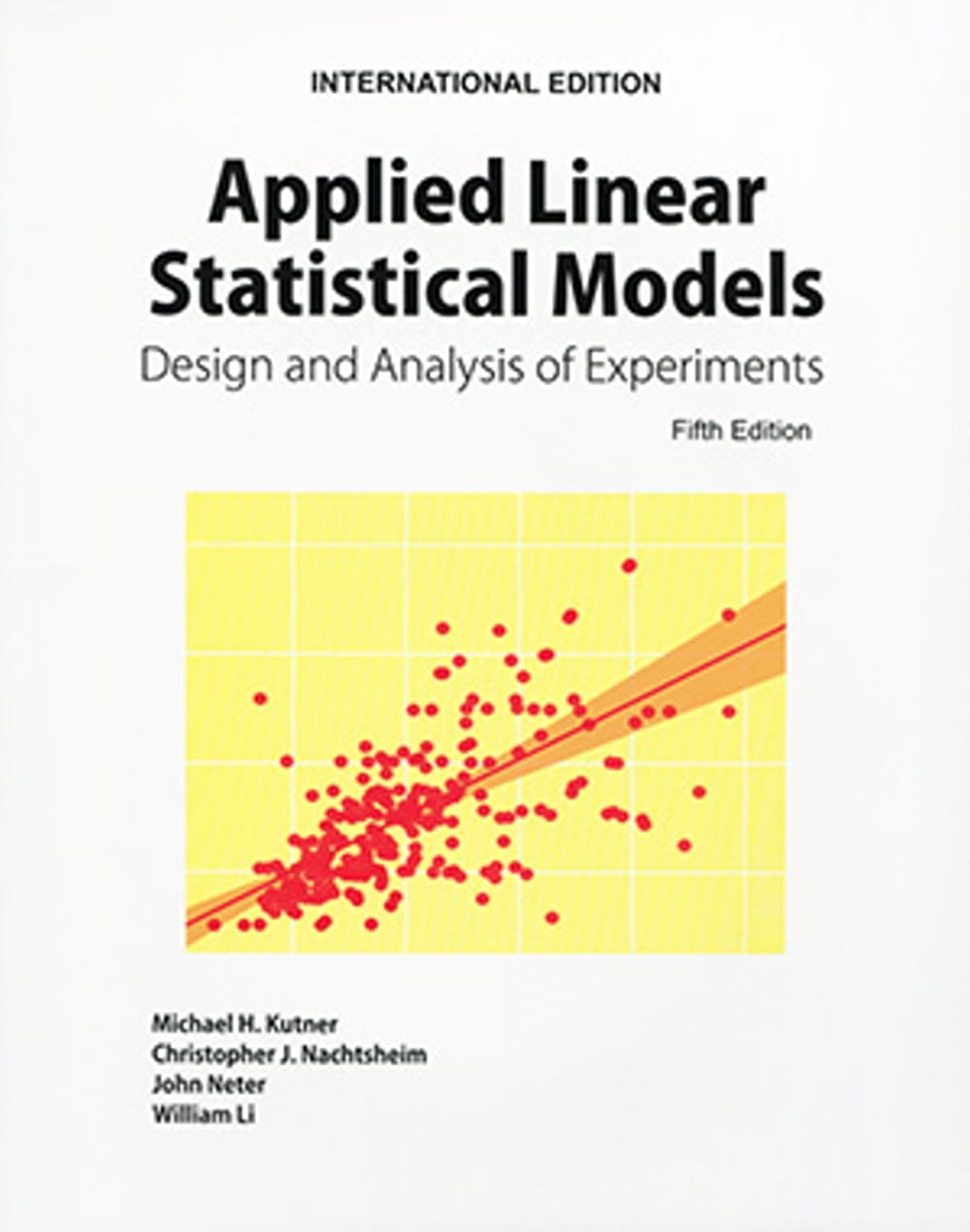{itemname} {itemname}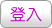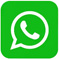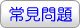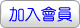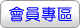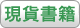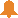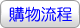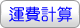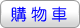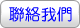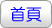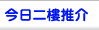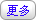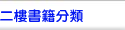Applied Linear Statistical Models：Applied Linear Regression Models（5版）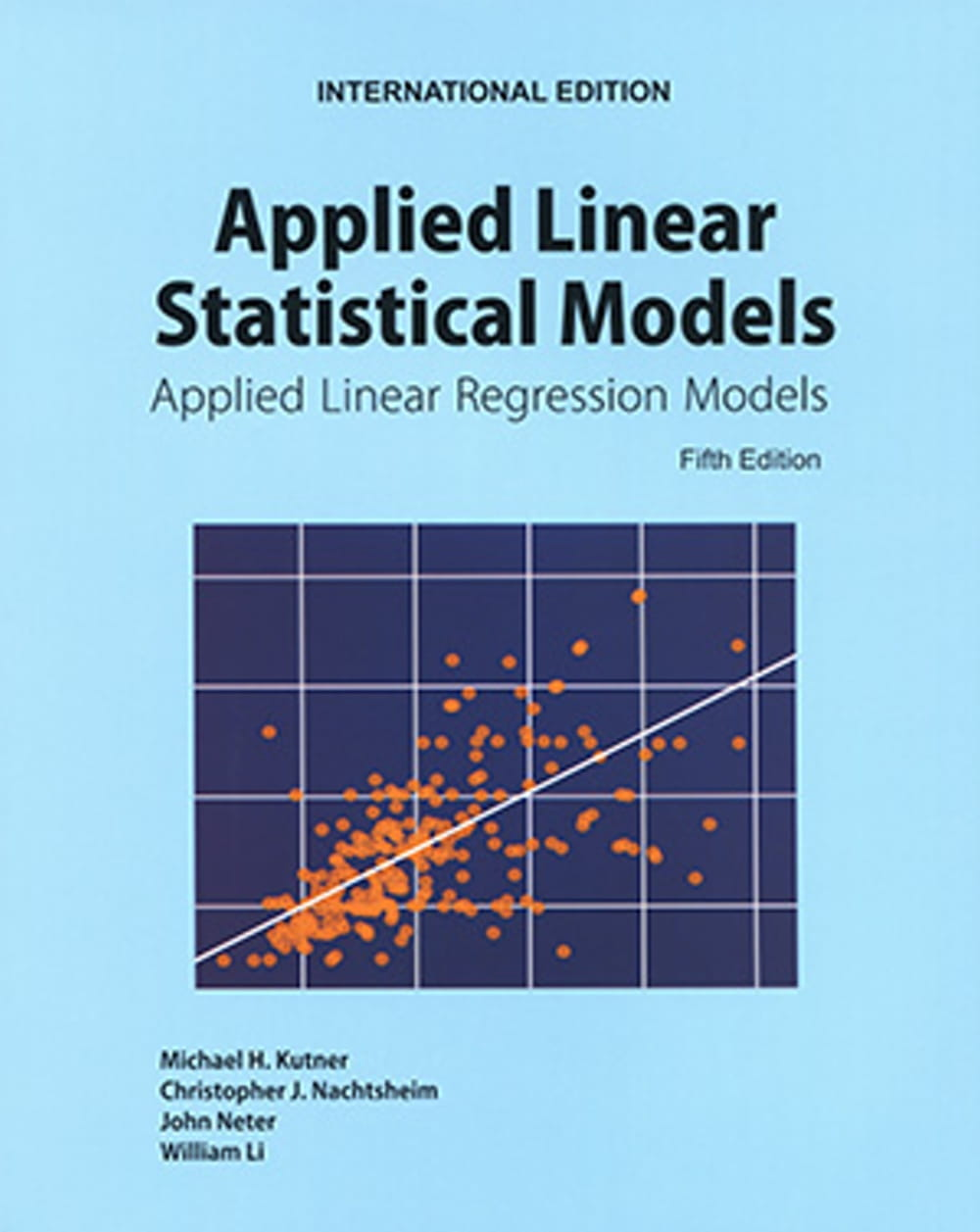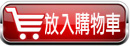沒有庫存 訂購需時10-14天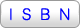9789863414179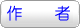Michael H. Kutner,Christopher J. Nachtsheim,John Neter,William Li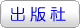華泰文化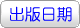2019年9月04日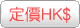427.00  元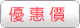HK\$ 405.65省下 \$21.35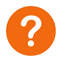ISBN：9789863414179
• 規格：平裝 / 740頁 / 18.6 x 23.2 x 3.5 cm / 普通級 / 單色印刷 / 5版
• 出版地：台灣

• [ 尚未分類 ]

1. Added material on important techniques for data mining, including regression trees and neural network models in Chapters 11 and 13.

2. The Chapter on logistic regression (Chapter 14) has been extensively revised and expanded to include a more thorough treatment of logistic, probit, and complementary log-log models, logistic regression residuals, model selection, model assessment, logistic regression diagnostics, and goodness of fit tests. We have also developed new material on polytomous (multicategory) nominal logistic regression models and polytomous ordinal logistic regression models.

3. We have expanded the discussion of model selection methods and criteria. The Akaike information criterion and Schwarz Bayesian criterion have been added, and a greater emphasis is placed on the use of cross-validation for model selection and validation.

4. New open ended Cases based on data sets from business, health care, and engineering are included. Also, many problem data sets have been updated and expanded.

5. The text includes a CD with all data sets and the Student Solutions manual in PDF. In addition a new supplement, SAS and SPSS Program Solutions by Replogle and Johnson is available for the Fifth Edition.

PART I: SIMPLE LINEAR REGRESSION

Ch 1 Linear Regression with One Predictor Variable

Ch 2 Inferences in Regression and Correlation Analysis

Ch 3 Diagnostics and Remedial Measures

Ch 4 Simultaneous Inferences and Other Topics in Regression Analysis

Ch 5 Matrix Approach to Simple Linear Regression Analysis

PART II: MULTIPLE LINEAR REGRESSION

Ch 6 Multiple Regression I

Ch 7 Multiple Regression II

Ch 8 Regression Models for Quantitative and Qualitative Predictors

Ch 9 Building the Regression Model I: Model Selection and Validation

Ch10 Building the Regression Model II: Diagnostics

Ch11 Building the Regression Model III: Remedial Measures

Ch12 Autocorrelation in Time Series Data

PART III: NONLINEAR REGRESSION

Ch13 Introduction to Nonlinear Regression and Neural Networks

Ch14 Logistic Regression, Poisson Regression, and Generalized Linear Models

 其 他 著 作 1. Applied Linear Statistical Models：Design and Analysis of Experiments（5版）